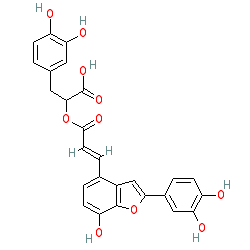=====================================================SMILES: C1=C(OC2=C1C(=CC=C2O)\C=C\C(OC(CC3=CC(=C(O)C=C3)O)C(=O)O)=O)C4=CC(=C(O)C=C4)O
InChIKey: GCJWPRRNLSHTRY-XBXARRHUSA-N

–EOF–